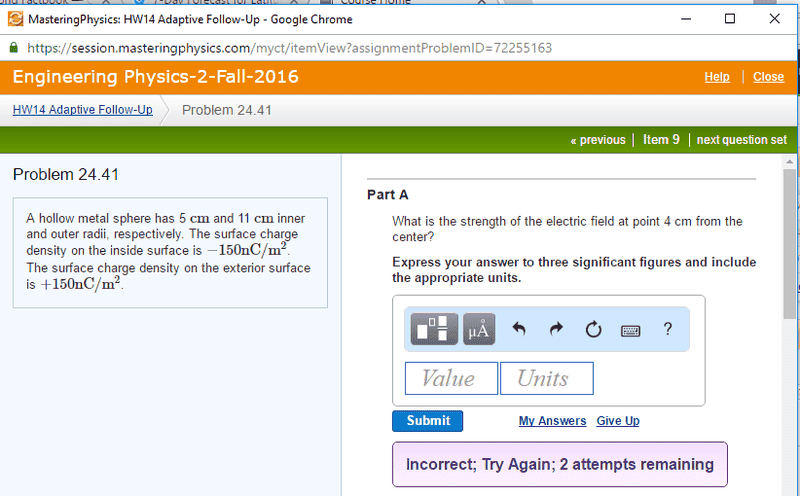# What is the Electric Field inside this charged sphere?

## Homework Statement

Let me just put this here:
http://i.imgur.com/dgcWAC3.png.

## Homework Equations

E_flux=EA=(q_encl)/(permittivity)
Area=4pir^2

## The Attempt at a Solution

Whenever I manipulate the above equations, I get a term of the form R/r, which implies, R being 5 cm, and r being the radius inside. This implies that the field goes to infinity at the center, when I'm pretty sure by spherical symmetry it is 0!